Anzeige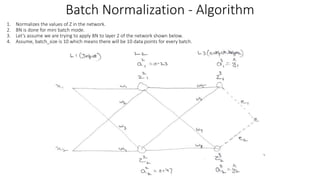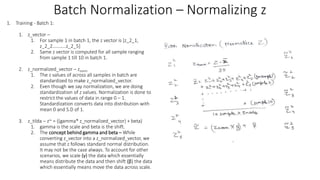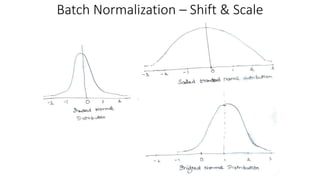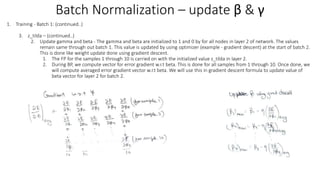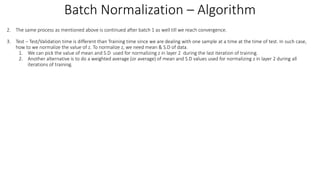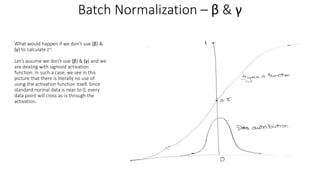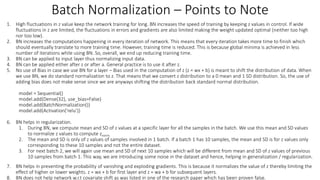1 von 7
Anzeige

### Batch Normalization

1. 1. Normalizes the values of Z in the network. 2. BN is done for mini batch mode. 3. Let’s assume we are trying to apply BN to layer 2 of the network shown below. 4. Assume, batch_size is 10 which means there will be 10 data points for every batch. Batch Normalization - Algorithm
2. 1. Training - Batch 1: 1. z_vector – 1. For sample 1 in batch 1, the z vector is [z_2_1, z_2_2………..z_2_5] 2. Same z vector is computed for all sample ranging from sample 1 till 10 in batch 1. 2. z_normalized_vector – znorm 1. The z values of across all samples in batch are standardized to make z_normalized_vector. 2. Even though we say normalization, we are doing standardization of z values. Normalization is done to restrict the values of data in range 0 – 1. Standardization converts data into distribution with mean 0 and S.D of 1. 3. z_tilda – z~ = ((gamma* z_normalized_vector) + beta) 1. gamma is the scale and beta is the shift. 2. The concept behind gamma and beta – While converting z_vector into a z_normalized_vector, we assume that z follows standard normal distribution. It may not be the case always. To account for other scenarios, we scale (γ) the data which essentially means distribute the data and then shift (β) the data which essentially means move the data across scale. Batch Normalization – Normalizing z
3. Batch Normalization – Shift & Scale
4. 1. Training - Batch 1: (continued..) 3. z_tilda – (continued..) 2. Update gamma and beta - The gamma and beta are initialized to 1 and 0 by for all nodes in layer 2 of network. The values remain same through out batch 1. This value is updated by using optimizer (example - gradient descent) at the start of batch 2. This is done like weight update done using gradient descent. 1. The FP for the samples 1 through 10 is carried on with the initialized value z_tilda in layer 2. 2. During BP, we compute vector for error gradient w.r.t beta. This is done for all samples from 1 through 10. Once done, we will compute averaged error gradient vector w.r.t beta. We will use this in gradient descent formula to update value of beta vector for layer 2 for batch 2. Batch Normalization – update β & γ
5. 2. The same process as mentioned above is continued after batch 1 as well till we reach convergence. 3. Test – Test/Validation time is different than Training time since we are dealing with one sample at a time at the time of test. In such case, how to we normalize the value of z. To normalize z, we need mean & S.D of data. 1. We can pick the value of mean and S.D used for normalizing z in layer 2 during the last iteration of training. 2. Another alternative is to do a weighted average (or average) of mean and S.D values used for normalizing z in layer 2 during all iterations of training. Batch Normalization – Algorithm
6. Batch Normalization – β & γ What would happen if we don’t use (β) & (γ) to calculate z~. Let’s assume we don’t use (β) & (γ) and we are dealing with sigmoid activation function. In such a case, we see in this picture that there is literally no use of using the activation function itself. Since standard normal data is near to 0, every data point will cross as-is through the activation.
7. 1. High fluctuations in z value keep the network training for long. BN increases the speed of training by keeping z values in control. If wide fluctuations in z are limited, the fluctuations in errors and gradients are also limited making the weight updated optimal (neither too high nor too low). 2. BN increases the computations happening in every iteration of network. This means that every iteration takes more time to finish which should eventually translate to more training time. However, training time is reduced. This is because global minima is achieved in less number of iterations while using BN. So, overall, we end up reducing training time. 3. BN can be applied to input layer thus normalizing input data. 4. BN can be applied either after z or after a. General practice is to use it after z. 5. No use of Bias in case we use BN for a layer – Bias used in the computation of z (z = wx + b) is meant to shift the distribution of data. When we use BN, we do standard normalization to z. That means that we convert z distribution to a 0 mean and 1 SD distribution. So, the use of adding bias does not make sense since we are anyways shifting the distribution back standard normal distribution. model = Sequential() model.add(Dense(32), use_bias=False) model.add(BatchNormalization()) model.add(Activation('relu’)) 6. BN helps in regularization. 1. During BN, we compute mean and SD of z values at a specific layer for all the samples in the batch. We use this mean and SD values to normalize z values to compute znorm. 2. The mean and SD is only of z values of samples involved in 1 batch. If a batch 1 has 10 samples, the mean and SD is for z values only corresponding to these 10 samples and not the entire dataset. 3. For next batch 2, we will again use mean and SD of next 10 samples which will be different from mean and SD of z values of previous 10 samples from batch 1. This way, we are introducing some noise in the dataset and hence, helping in generalization / regularization. 7. BN helps in preventing the probability of vanishing and exploding gradients. This is because it normalizes the value of z thereby limiting the effect of higher or lower weights. z = wx + b for first layer and z = wa + b for subsequent layers. 8. BN does not help network w.r.t covariate shift as was listed in one of the research paper which has been proven false. Batch Normalization – Points to Note

### Hinweis der Redaktion

1. Why BN is not applied in batch or stochastic mode?
2. Why BN is not applied in batch or stochastic mode?
3. Why BN is not applied in batch or stochastic mode?
4. Why BN is not applied in batch or stochastic mode?
5. Why BN is not applied in batch or stochastic mode?
6. Why BN is not applied in batch or stochastic mode?
7. Why BN is not applied in batch or stochastic mode?
Anzeige